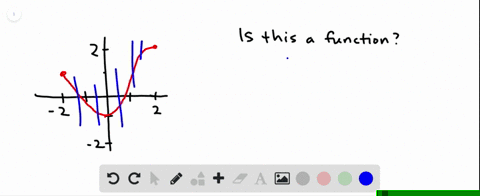Enroll in one of our FREE online STEM summer camps. Space is limited so join now!View Summer Courses### Determine whether the curve is the graph of a fun…

01:11Oregon State University

Need more help? Fill out this quick form to get professional live tutoring.

Get live tutoring
Problem 7

Determine whether the curve is the graph of a function of x. If it is, state the domain and range of the function.

No, the curve is not the graph of a function because a vertical line intersects the curve more than once. Hence, the curve fails the Vertical Line Test.

## Discussion

You must be signed in to discuss.

## Video Transcript

okay. Is this the graph of a function? Well, remember that a function must pass the vertical line test, which means that for every X value there's only one y value. So if you pass a vertical line through, it can only pass through one point. This vertical line passes through only one point, and so does this one. However, this vertical line passes through multiple points, as does this one and this one. And even though there are some vertical lines that pass through only one point, if there exists any vertical lines that pass through multiple points, it's not a function.